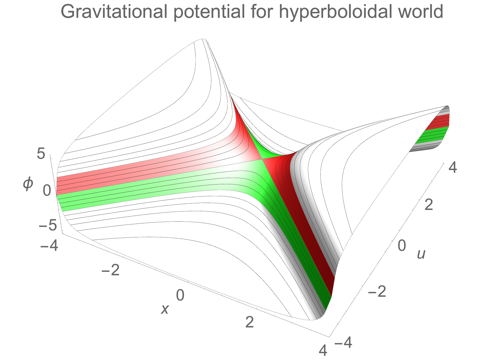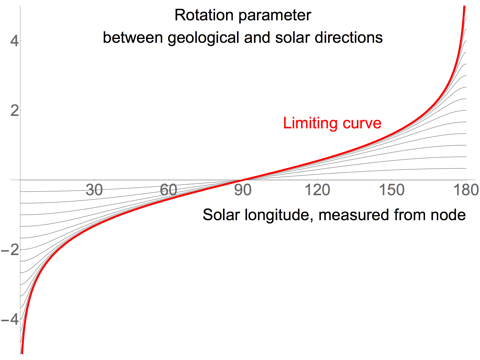# Dichronauts

## The World of Dichronauts [Extra]

This page contains some extra mathematical details to supplement the main page on this topic. In everything that follows, we will give the three dimensions of space the coordinates x, y and u, where x and y are ordinary, “space-like” dimensions, and u is a “time-like” dimension. This means that we need to use a metric g which defines the dot product between vectors as:

v · w = g(v, w) = vx wx + vy wyvu wu

and gives the squared magnitude of a vector as:

v · v = g(v, v) = vx2 + vy2vu2

### The Gravitational Field of a Hyperboloidal World

[This supplements the section Gravity, and the Shape of the World on the main page, and should be read in conjunction with that.]

In our universe, the gravitational potential φ obeys Poisson’s equation:

2 φ = 4 π G ρ

where ρ is the density of matter and G is the gravitational constant. To understand what this is saying, we can write the Laplacian operator2 as:

2 φ = ∇ · (∇φ)

Since the gradient ∇φ is the opposite of the gravitational acceleration, the Laplacian ∇2 φ is proportional to the divergence of the acceleration. So Poisson’s equation for gravity links the density of matter with the divergence of the gravitational “lines of force” — essentially, it is saying that lines of force only end in matter, and the density of the endpoints is proportional to the density of matter.

In the Dichronauts universe, the potential obeys essentially the same equation, but because the dot product now has a minus sign for the time-like coordinate, we have a different sign for that coordinate:

 Equation for gravitational potential ∂x2 φ + ∂y2 φ – ∂u2 φ = 4 π G ρ Dichronauts universe ∂x2 φ + ∂y2 φ + ∂z2 φ = 4 π G ρ Our universe

The gravitational potential that corresponds to an infinite hyperboloidal distribution of matter is given below. In the two kinds of empty region outside the hyperboloids the potential consists of one term that is inversely proportional to the distance from the centre, plus a constant. For the interior, the potential is just proportional to the squared distance from the centre (interpreting that phrase a bit loosely, where we allow it to be negative in places.)

For comparison, we also give the potential for a spherical world in our own universe. Here, we have followed the convention that the potential goes to zero at infinity. In the hyperboloidal case, the result is more symmetrical if we make a different choice: having a potential of zero at the centre of the world. All of these choices are arbitrary, though; adding an overall constant to the gravitational potential makes no difference to the gravitational force, which is associated with the gradient of the potential.

Gravitational potential for hyperboloidal world
D=x2 + y2u2
φ(D) =
 2 π G ρ R2 (1 – 2R/[3√D]) D ≥ R2 (outside red hyperboloid) (2/3) π G ρ D –R2 < D < R2 (interior) –2 π G ρ R2 (1 – 2R/[3√(–D)]) D ≤ –R2 (outside green hyperboloids)
Dichronauts universe
Gravitational potential for spherical world
Sphere of radius R, density ρ
D=x2 + y2 + z2
φ(D) =
 –(4/3) π G ρ R3 / √D D ≥ R2 (exterior) (2/3) π G ρ (D – 3 R2) 0 ≤ D < R2 (interior)
Our universeThe potential for the hyperboloidal world is plotted in the image on the right, using the values R=1, ρ=1, G=1. The plot is for a slice in the x-u plane, but if we rotated the slice around the u-axis by any angle, the potential would look exactly the same.

To find the gravitational acceleration, we take the opposite of the gradient of the potential, –∇φ, in the usual way, but then we need to convert the gradient to a vector by “raising an index” with the appropriate metric. In Euclidean space, the metric is just the identity matrix, so the components of the vector and the gradient are identical, but in the Dichronauts space with one time-like dimension, the metric has entries of 1,1,–1 along the diagonal, so the acceleration vector has its time-like u component of the opposite sign to the gradient.

The change of sign we get from that formal derivation agrees with the intuitive idea that objects that lie in the time-like direction from the centre of the world will “roll uphill” in the direction of an increasing potential, instead of the usual behaviour of moving in the direction of decreasing potential. The result is that the gravitational acceleration at any location points directly towards the centre of the world.

Gravitational acceleration for hyperboloidal world
a=– (∂xφ, ∂yφ, –∂uφ)
D=x2 + y2u2
a(x, y, u) =
 –(4/3) π G ρ R3 / √(D3) (x, y, u) D ≥ R2 (outside red hyperboloid) –(4/3) π G ρ (x, y, u) –R2 < D < R2 (interior) –(4/3) π G ρ R3 / √(–D3) (x, y, u) D ≤ –R2 (outside green hyperboloids)
Dichronauts universe
Gravitational acceleration for spherical world
Sphere of radius R, density ρ
a=– (∂xφ, ∂yφ, ∂zφ)
D=x2 + y2 + z2
a(x, y, z) =
 –(4/3) π G ρ R3 / √(D3) (x, y, z) D ≥ R2 (exterior) –(4/3) π G ρ (x, y, z) 0 ≤ D < R2 (interior)
Our universe

### Solar vs Geological Coordinates

[This supplements the section The Grain of the Land, and the Migration on the main page, and should be read in conjunction with that.]

As the world rotates relative to the plane of the sun’s orbit, we can describe the midwinter circle (the circle on the surface of the world directly below the sun’s orbit) in Cartesian geological coordinates (x, y, u) as:

M(θ) = R (sin(θ) cosh(λ), cos(θ), –sin(θ) sinh(λ))

Here R is the radius of the world, and we have chosen geological coordinates such that the axis of rotation is the y-axis. The solar longitude, θ, measures the angle around the midwinter circle from the node at (0,R,0). The parameter λ measures how far the world has rotated, from the time when the plane of the sun’s orbit coincided with the equatorial plane in geological coordinates, u=0.

From M(θ), we can find a unit vector that points along the midwinter circle at any given longitude, giving a direction that we will call “solar east”:

SolarEast(θ) = ∂θ (M(θ) / R) = (cos(θ) cosh(λ), –sin(θ), –cos(θ) sinh(λ))

Now, at any point (x, y, u) on the one-sheeted hyperboloid that comprises part of the surface of the world, we can find unit vectors in the directions “geological east” and “geological north”:

GeoEast(x, y, u) = (y, –x, 0) / √(x2 + y2)
GeoNorth(x, y, u) = (u x, u y, x2 + y2) / √[(x2 + y2)(x2 + y2u2)]

The formula for GeoEast would actually work just as well on the surface of the Earth. It is clearly a unit vector, with no north-south component, and it is orthogonal to the vector from the centre of the world to the point (x, y, u). The formula for GeoNorth is also a unit vector (using the metric for the Dichronauts universe, where u is time-like), and it is orthogonal to both GeoEast and (x, y, u).

We can rewrite the direction SolarEast in terms of the geological directions GeoEast and GeoNorth:

SolarEast(θ) = [cosh(λ) GeoEast(M(θ)) – sinh(λ) cos(θ) GeoNorth(M(θ))] / √[1 + sinh(λ)2 sin(θ)2]We can then quantify the amount by which the geological and solar directions differ by taking the inverse hyperbolic sine of the geological north component of solar east:

μλ(θ) = arcsinh(–sinh(λ) cos(θ) / √[1 + sinh(λ)2 sin(θ)2])

A hyperbolic rotation with this parameter μλ(θ) will take geological east into solar east. If θ=0, i.e. if we look at the rotation at the node, this becomes simply: μλ(0) = –λ.

The limit as λ → ∞ is:

μ(θ) = arcsinh(–cos(θ) / |sin(θ)|)

The image on the right is a plot of μλ(θ) for various values of λ, in grey, along with the limiting curve μ(θ) in red. The existence of this limiting curve means that the rotation parameter at solar longitudes not too far from 90 degrees is bounded, and so the problems caused by a large disparity between the geological and solar directions will only occur closer to the nodes.Dichronauts / The World of Dichronauts [Extra] / created Sunday, 11 December 2016